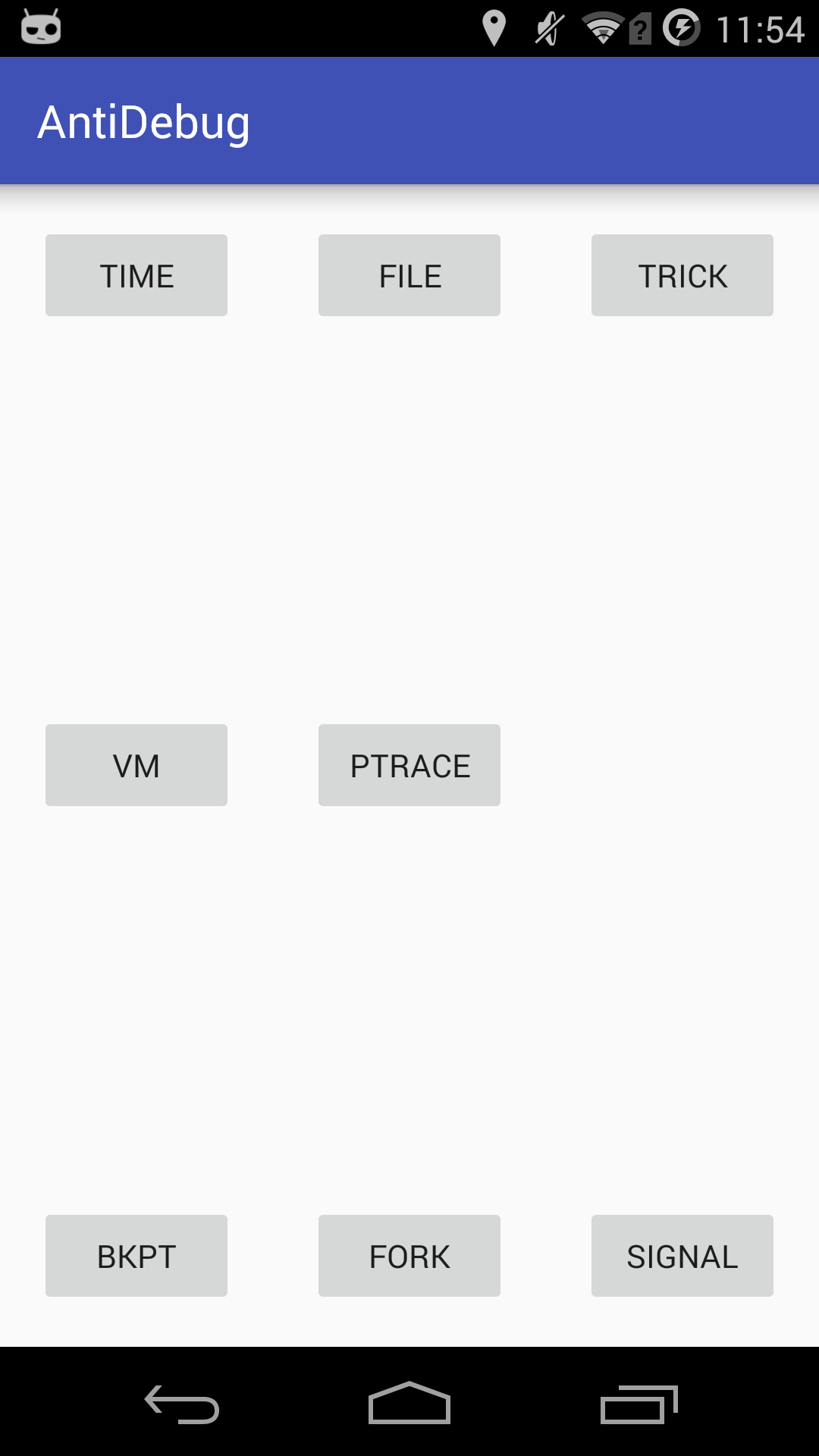# Android反调试技术整理与实践

Posted by GToad on June 25, 2017

## 基于时间的检测

``````extern "C"
JNIEXPORT jstring

JNICALL
JNIEnv *env,
jobject /* this */) {
long start,end;
std::string hello = "Hello from time";
start = clock();
...
（部分代码逻辑）
...
end = clock();
if(end-start>10000){
hello = "Debug from time";
}
return env->NewStringUTF(hello.c_str());
}
``````

## 基于文件的检测

1. /proc/pid/status 和 /proc/pid/task/pid/status：普通状态下，TracerPid这项应该为0；调试状态下为调试进程的PID。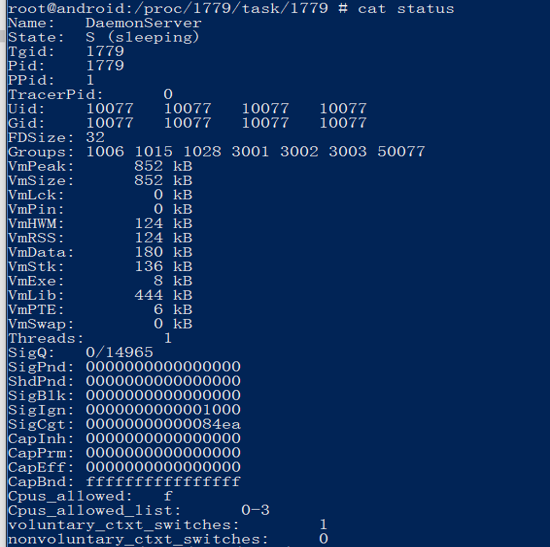2. /proc/pid/stat 和 /proc/pid/task/pid/stat：调试状态下，括号后面的第一个字母应该为t。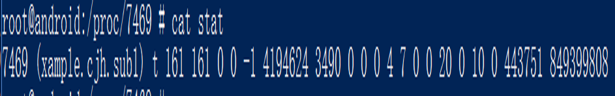3. /proc/pid/wchan 和 /proc/pid/task/pid/wchan：调试状态下，里面内容为ptrace_stop。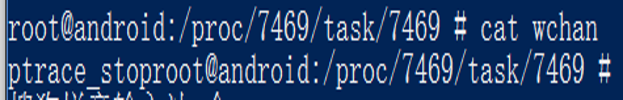``````extern "C"
JNIEXPORT jstring

JNICALL
JNIEnv *env,
jobject /* this */) {
std::string hello;
std::stringstream stream;
int pid = getpid();
int fd;
stream << pid;
stream >> hello;
hello = "/proc/" + hello + "/status";
//LOGI(hello);
char* pathname = new char;
strcpy(pathname,hello.c_str());
char* buf = new char;
int flag = O_RDONLY;
fd = open(pathname, flag);
char* c;
char* tra = "TracerPid";
c = strstr(buf, tra);
char* d;
d = strstr(c,"\n");
int length = d-c;
strncpy(buf,c+11,length-11);
buf[length-11]='\0';
hello = buf;
if (strcmp(buf,"0")){
hello = "Debug from file";
}
else{
hello = "Hello from file";
}
close(fd);

return env->NewStringUTF(hello.c_str());
}
``````

## Dalvik虚拟机内部相关字段

Android Java虚拟机结构中也保存了是否被调试的相关数据。Android 5.0以前的系统版本中可以通过调用java.lang.Object->dalvik.system.VMDebug->Dalvik_dalvik_system_VMDebug_isDebuggerConnected()来获得结果。之后的版本中改成了android.os.Debug类下的isDebuggerConnected()方法。 由于是Java层的系统调用，所以相比于Native层，本方法会更容易被发现，且被Hook篡改返回值也会更简单。

``````if(android.os.Debug.isDebuggerConnected()){
Toast.makeText(context, "Debug from vm",Toast.LENGTH_LONG).show();
}
else{
Toast.makeText(context, "Hello from vm",Toast.LENGTH_LONG).show();
}
``````

## ptrace

``````extern "C"
JNIEXPORT jstring

JNICALL
JNIEnv *env,
jobject /* this */) {
int check = ptrace(PTRACE_TRACEME,0 ,0 ,0);
LOGI("ret of ptrace : %d",check);
...
部分逻辑代码
...
std::string hello = "Hello from ptrace";
if(check != 0){
hello = "Debug from ptrace";
}
return env->NewStringUTF(hello.c_str());
}
``````

## 断点扫描

IDA等调试器在调试时候的原理是向断点地址插入breakpoint汇编指令，而把原来的指令暂时备份到别处。因此，本方法通过扫描自身so的代码部分中是否存在breakpoint指令即可。

1. Arm：0x01，0x00，0x9f，0xef
2. Thumb16：0x01，0xde
3. Thumb32：0xf0，0xf7，0x00，0xa0

``````bool checkBreakPoint ()
{
__android_log_print(ANDROID_LOG_INFO,"JNI","13838438");
int i, j;
Elf32_Ehdr *elfhdr;
Elf32_Phdr *ph_t;

if (base == 0) {
return false;
}
__android_log_print(ANDROID_LOG_INFO,"JNI","13838439");

elfhdr = (Elf32_Ehdr *) base;

for (i = 0; i < elfhdr->e_phnum; i++) {

if ( !(ph_t->p_flags & 1) ) continue;
offset += sizeof(Elf32_Ehdr) + sizeof(Elf32_Phdr) * elfhdr->e_phnum;

char *p = (char*)offset;
for (j = 0; j < ph_t->p_memsz; j++) {
if(*p == 0x01 && *(p+1) == 0xde) {
LOGI ("Find thumb bpt %p", p);
return true;
} else if (*p == 0xf0 && *(p+1) == 0xf7 && *(p+2) == 0x00 && *(p+3) == 0xa0) {
LOGI ("Find thumb2 bpt %p", p);
return true;
} else if (*p == 0x01 && *(p+1) == 0x00 && *(p+2) == 0x9f && *(p+3) == 0xef) {
LOGI ("Find arm bpt %p", p);
return true;
}
p++;
}
}
return false;
}
``````

## 信号处理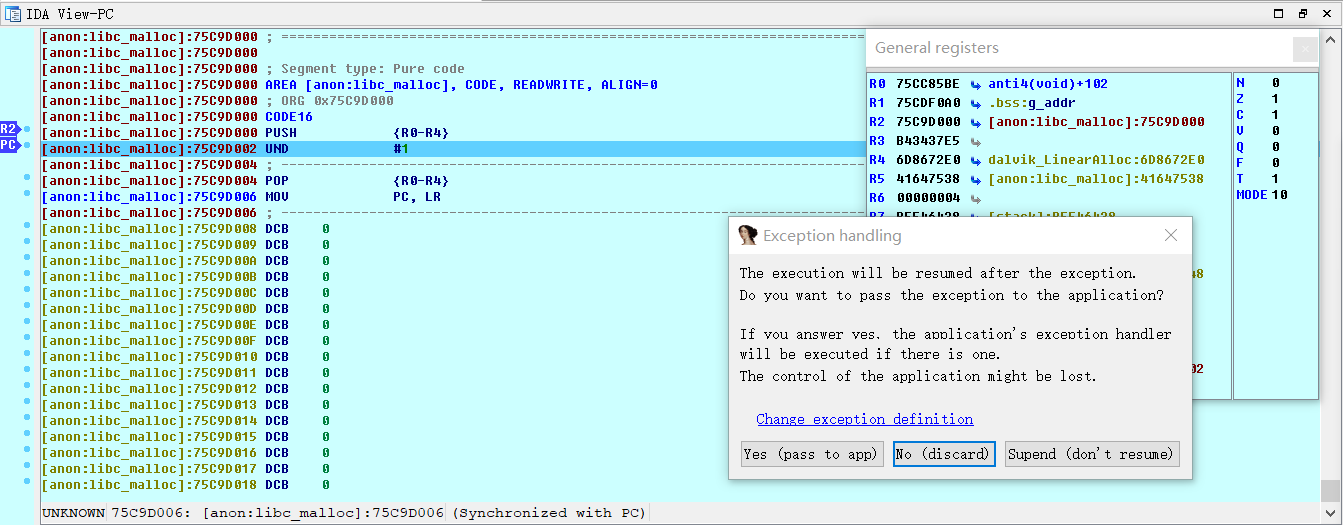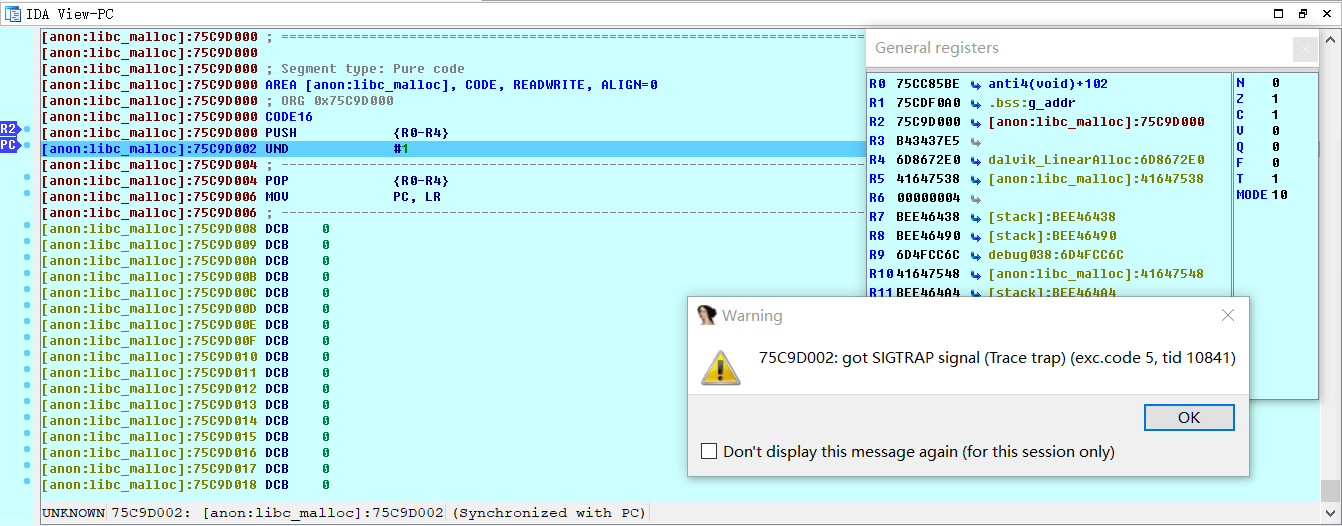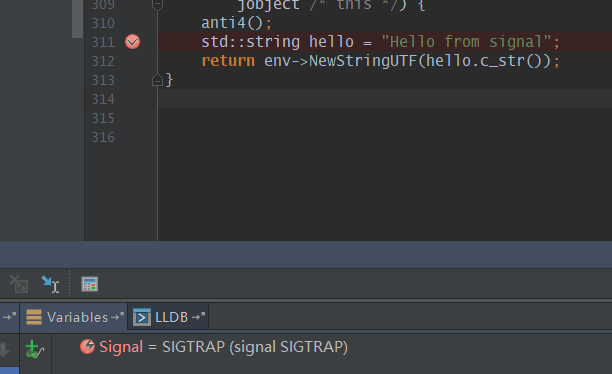``````// 跳转到bkpt陷阱处：

__asm__("push {r5}\n\t"
"push {r0-r4,lr}\n\t"
"mov r0,pc\n\t"
"mov lr,r0\n\t"
"mov pc,%0\n\t"
"pop {r0-r5}\n\t"
"mov lr,r5\n\t"
"pop {r5}\n\t"
:
:);

// bkpt陷阱处：

push {r0-r4}
breakpoint——thumb16{0x01,0xde} thumb32{0xf0,0xf7,0x00,0xa0} arm32{0x01,0x00,0x9f,0xef}
pop {r0-r4}
mov pc, lr

// signal handler处：

void my_sigtrap(int sig){
LOGI("my_sigtrap\n");

char change_bkp[] = {0x00,0x46}; //mov r0,r0
LOGI("chang bpk to nop\n");

}
``````

## 调试器的错误理解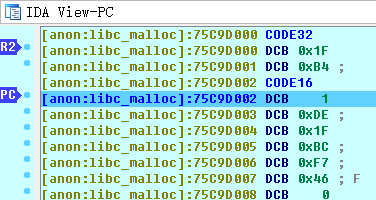## 多进程/线程

1. 基于文件的检测
2. 断点扫描
3. 基于套路的检测
4. 基于时间的检测（极少）
5. Dalvik虚拟机内部相关字段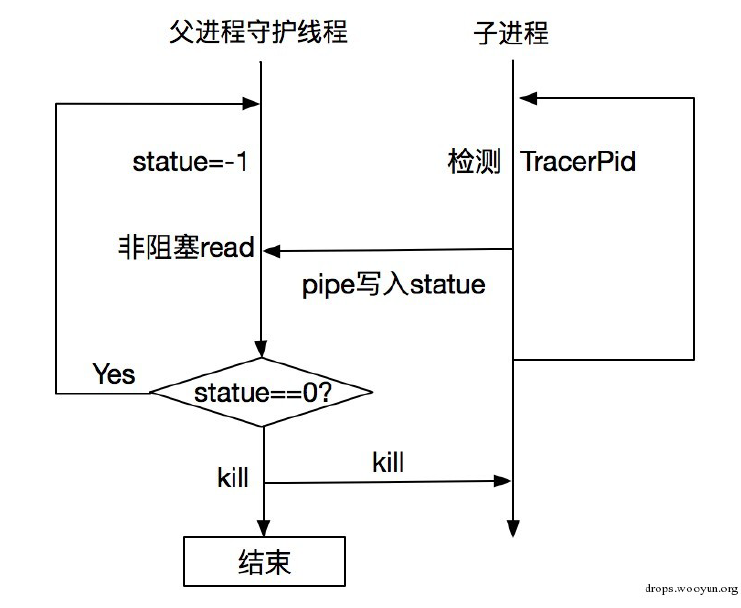``````int pipefd;
int childpid;

int statue=-1,alive=1,count=0;

close(pipefd);

break;
sleep(1);

//这里改为非阻塞
fcntl(pipefd, F_SETFL, O_NONBLOCK); //enable fd的O_NONBLOCK

while(true) {

LOGI("pip--> statue = %d", statue);
sleep(1);

LOGI("pip--> statue2 = %d", statue);
if (statue != 0) {
kill(childpid,SIGKILL);
kill(getpid(), SIGKILL);
return NULL;
}
statue = -1;
}
}

void anti3(){
int pid,p;
FILE *fd;
char filename[MAX];
char line[MAX];

pid = getpid();
sprintf(filename,"/proc/%d/status",pid);// 读取proc/pid/status中的TracerPid
p = fork();
if(p==0) //child
{
LOGI("Child");
close(pipefd); //关闭子进程的读管道
int pt,alive=0;
pt = ptrace(PTRACE_TRACEME, 0, 0, 0); //子进程反调试
while(true)
{
fd = fopen(filename,"r");
while(fgets(line,MAX,fd))
{
if(strstr(line,"TracerPid") != NULL)
{
LOGI("line %s",line);
int statue = atoi(&line);
LOGI("########## tracer pid:%d", statue);
write(pipefd,&statue,4);//子进程向父进程写 statue值

fclose(fd);

if(statue != 0)
{
LOGI("########## tracer pid:%d", statue);
return ;
}

break;
}
}
sleep(1);

}
}else{
LOGI("Father");
childpid = p;
}
}

extern "C"
JNIEXPORT jstring

JNICALL
JNIEnv *env,
jobject /* this */) {
std::string hello = "Hello from fork";
pipe(pipefd);
LOGI("Start");
anti3();
return env->NewStringUTF(hello.c_str());
}
``````

## 样例APK

1. `TIME`按钮：基于时间的检测，未发现调试返回”Hello from time”,发现调试返回”Debug from time”
2. `FILE`按钮：基于文件的检测，未发现调试返回”Hello from file”,发现调试返回”Debug from file”
3. `VM`按钮：Dalvik虚拟机内部相关字段，未发现调试返回”Hello from vm”,发现调试返回”Debug from vm”
4. `PTRACE`按钮：基于ptrace的检测，未发现调试返回”Hello from ptrace”,发现调试返回”Debug from ptrace”
5. `BKPT`按钮：断点扫描，未发现调试返回”Hello from bkpt”,发现调试返回”Debug from bkpt”。
6. `FORK`按钮：多进程/线程，开启检测后会立刻返回”Hello from fork”,发现调试后直接杀死本APP。本按钮按下后效果持续，建议最后尝试。
7. `SIGNAL`按钮：调试器的错误理解+信号处理，未发现调试返回”Hello from signal”,发现调试后前者会让调试崩溃，后者会让调试卡死。